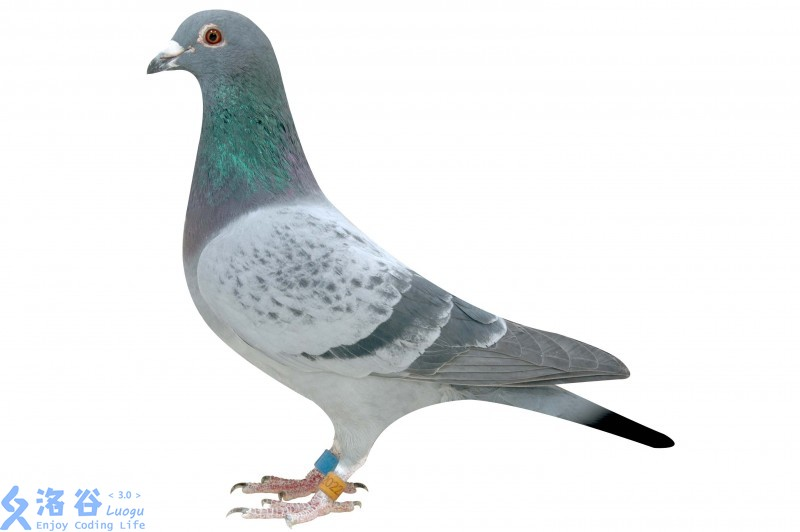# ShineEternal的小书屋# ShineEternal的小书屋

https://blog.csdn.net/kkkksc03

### 鸽巢原理及其扩展——Ramsey定理

posted on 2018-08-23 10:11:55 | under 日报 |

# 第一部分：鸽巢原理a数组：but 我也很重要

$：我好像也出现不少次 **以上纯属灌水** ------------ 文章简叙：鸽巢原理对初赛时的问题求解以及复赛的数论题目都有启发意义。直接的初赛考察一般在提高组出现。相当于抽屉。 别名：鸽笼原理。狄利克雷抽屉原理。 **最简单的一种形式**：有$ m+1 $只鸽子，$ m $个笼子，那么至少有一个笼子有至少两只鸽子。当然，换个角度来说：有$ m-1 $只鸽子，$ m $个笼子，那么至少有一个笼子是空的。 ![luogu](https://cdn.luogu.com.cn/upload/pic/30163.png) **初级加强**：有$ m $个笼子，$ km+1 $只鸽子，那么至少有一个笼子有至少$ k+1 $只鸽子。 **高级加强**：令 -$ a_1,a_2,a_3...a_m $- 为正整数。 -$ if $我们将 -$ a_1+a_2+a_3+...+a_n-n+1 $- 个鸽子放入$ n $个笼子里，$ then $， ||第一个笼子至少有$ a_1 $只鸽子||第二个笼子至少有$ a_2 $只鸽子||第三个笼子至少有$ a_3 $只鸽子||...||第$ m $个笼子至少有$ a_m $只鸽子 ------------ # 鸽巢原理的应用 **一位洛谷$ oier $要用$ 12 $周的时间准备$ CTSC $,为了练习，他每天至少要刷一题，因为题目有难度，他每星期刷题无法超过$ 13 $题。请你证明：存在连续的若干天期间，这位$ oier $恰好刷了$ 11 $题** 开始证明： 1.我们可以令$ a_1 $表示第一天所刷的题数，$ a_2 $表示前两天所刷的题数，$ a_3 $表示前三天所刷的题数.之后以此类推 2.而题目说，由于每天都要至少刷1题，所以数列 -$ a_1,a_2,a_3,a4,...,a{84} $- 严格递增。另有$ a1>=1 $.又每周最多刷13题，故$ a{84}<=1312=156 $. 3.因此又有： -$ 1<=a_1<a_2<a3<...<a{84}<=156 $. 4.同理， -$ a_1+11,a_2+11,a3+11,...,a{84}+11 $- 同样是一个严格递增序列。范围： -$ 12<=a_1+11<a_2+11<a3+11<...<a{84}+11<=167 $5.我们把两个序列合起来看： -$ a_1,a_2,a3,...,a{84},a_1+11,a_2+11,a3+11,...,a{84}+11 $- 一共$ 168 $个数。其中每一个数都是$ 1 $到$ 167 $之间的一个整数。 6.**根据鸽巢原理可得，其中必有两个数相等！！！** 7.既然 -$ a_1,a_2,a3,...,a{84} $- 中必然无相等的两个数， - 那么$ a_1+11,a_2+11,a3+11,...,a{84}+11 $- 中同理。那么，必然存在一个$ x $和一个$ y $,使得 -$ a_x=a_y+11 $; 8.从而得出结论：这个$ oier $在第 -$ y+1,y+2,y+3,...,x $- 天内一共刷了$ 11 $道题 ------------ # 应用二 **证明：在边长为$ 2 $的等边三角形中放上$ 5 $个点。则至少存在两个点，他们之间的距离小于等于$ 1 $.** ![Luogu](https://cdn.luogu.com.cn/upload/pic/30162.png) 1.我们先画出一个边长为$ 2 $的等边三角形。 2.然后把三条边中点两两相连。就形成了这张图。 3.那么根据鸽巢原理，必然有两个点在一个边长为$ 1 $的小三角形里。 4.而我们知道，边长为$ 1 $的等边三角形里处处距离都小于等于$ 1 $5.于是问题就解决了 ------------ # 应用三 **已知$ n+1 $个正整数，它们全都小于或等于$ 2n $，证明当中一定有两个数是互质的。** 1.要证明这个问题，我们就要利用一个互质的特性：两个相邻整数互质。 2.有了这个突破口，于是我们可以构造n个鸽巢，每一个里依次放入 -$ 1,2,3,...,2n $- 这2n个数中的两个数。 ![luogu](https://cdn.luogu.com.cn/upload/pic/30161.png) 3.也就是说，我们要在这其中取出$ n+1 $个数。 4.根据鸽巢原理，无论如何，我们都会抽空一个鸽巢。 5.一个鸽巢中的两个数肯定互质，所以问题就解决了。 **扒栗史**：匈牙利大数学家厄杜斯(PaulErdous,1913 - 1996) 向当年年仅$ 11 $岁的波萨(LouisPósa)提出这个问题，而小波萨思考了不足半分钟便能给出正确的答案。 ------------ **有趣的小（leng）知（xiao）识（hua）**： 山东高考$ 2017 $年有$ 54 $万人。而人的头发大约有$ 8-12 $万根。那么必然有两人的头发数量相同。 好了，现在来一道初赛真题收（dian）心(di): **【NOIP2010 提高组】记$ T $为一队列，初始时为空，现有n个总和不超过$ 32 $的正整数依次入队，如果无论这些数具体为什么值，都能找到一种出队的方式，使得存在某个时刻队列$ T $中的数之和恰好为$ 9 $，那么$ n $的最小值是_______________** 1.第一眼看到此题，蒟蒻就知道自己只能~~根据结果推过程~~了 2.刚开始看了一眼答案：$ 18 $. 3.于是就根据这个开始推导过程。我们可以令$ a_i $表示前$ i $个数的和，并约定：$ a_0=0 $. 4.题目要求求出最小的$ n $，使得存在$ 0<=x<y<=n $满足$ a_y=a_x+9 $; 5.于是我们可将$ a $数组看做鸽子，用不能同时取的一组（差为$ 9 $）的集合构造笼子， 6.构造方法如下：一共有$ n=18 $个集合按此方式选取： -$ {0,9}、{1,10}、{2,11}、...、{8,17}、{18,27}、{19,28}、{20,29}、...、{23,32}、{24}、{25}、{26} $。 7.由题意可知，我们一旦在某个集合中取了两个元素，$ then $一定存在某个时刻队列$ T $中数的总和恰好为$ 9 $. 8.于是由鸽巢原理，我们可以得知：$ n=18 $一定满足条件. **但是题目要让我们求出最小值，为了保险起见（都看答案了还保什么险）：** 1.我们还要证明一下$ n=17 $不可行。 2.然鹅我们只需要举出反例即可： - 1 1 1 1 1 1 1 1 10 1 1 1 1 1 1 1 1 3.说明：因为每到了$ 8 $个$ 1 $就被$ 10 $隔断，故不可行。 ------------ # 第二部分：Ramsey定理 **扒栗史**：此定理由Frank Plumpton Ramsey（弗兰克·普伦普顿·拉姆齐，$ 1903-1930\$）提出.

• 此定理有一个广为流传的例子：6 个人中至少存在3人相互认识或者相互不认识。

• 转换：该定理等价于证明这6个顶点的完全图的边，用红、蓝二色任意着色，必然至少存在一个红色边三角形，或蓝色边三角形